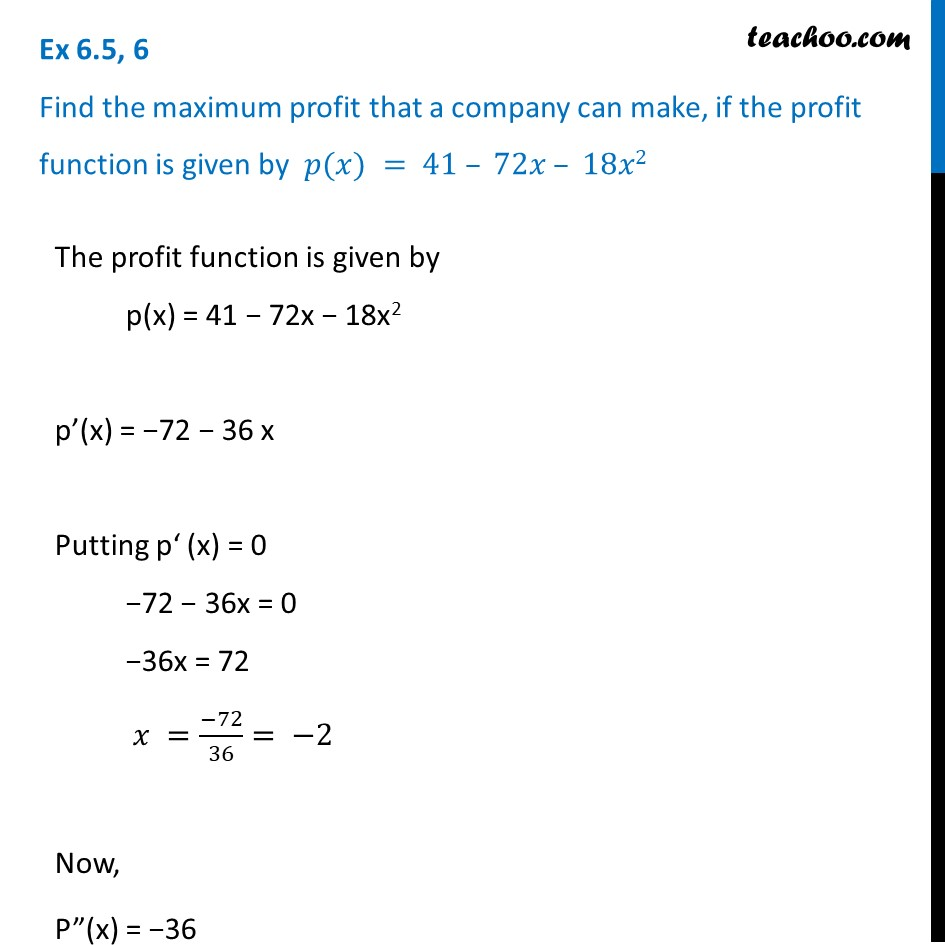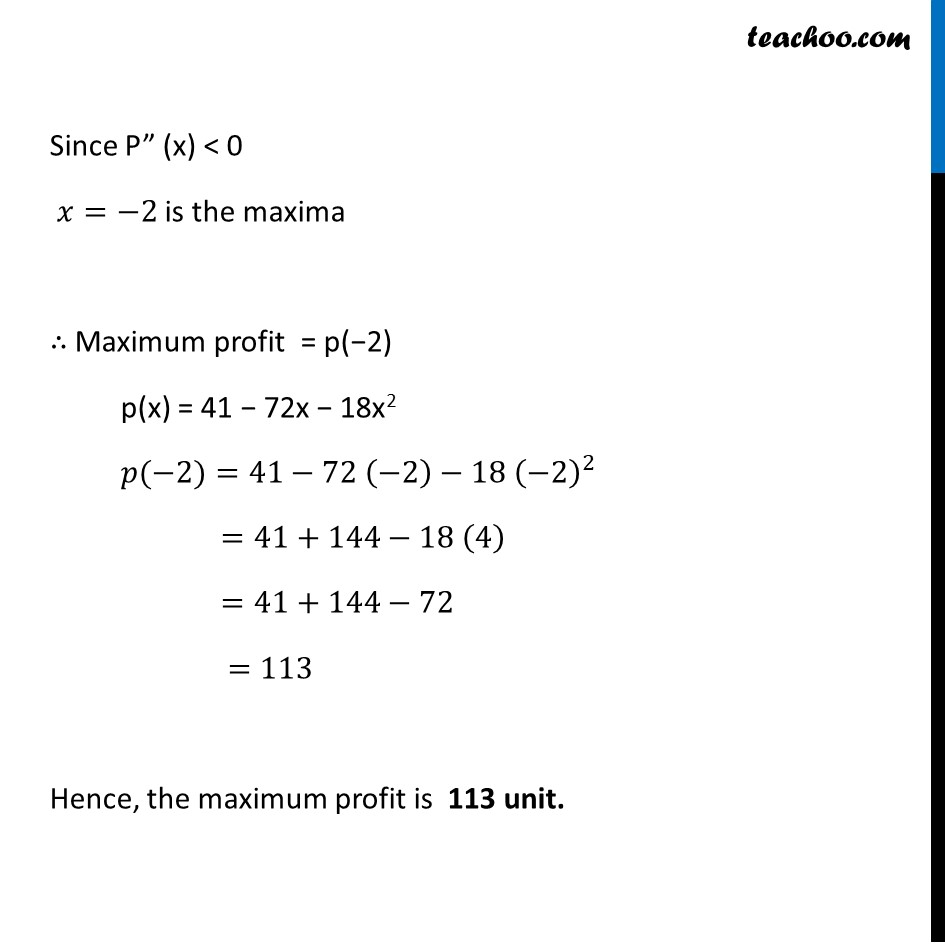Local maxima and minima

Chapter 6 Class 12 Application of Derivatives
Concept wiseLearn in your speed, with individual attention - Teachoo Maths 1-on-1 Class

### Transcript

Ex 6.3, 6 Find the maximum profit that a company can make, if the profit function is given by 𝑝(𝑥) = 41 – 72𝑥 – 18𝑥2The profit function is given by p(x) = 41 − 72x − 18x2 p’(x) = −72 − 36 x Putting p‘ (x) = 0 −72 − 36x = 0 −36x = 72 𝑥 =(−72)/36= −2 Now, P”(x) = −36 Since P” (x) < 0 𝑥=−2 is the maxima ∴ Maximum profit = p(−2) p(x) = 41 − 72x − 18x2 𝑝(−2)=41−72 (−2)−18 (−2)^2 =41+144−18 (4) =41+144−72 =113 Hence, the maximum profit is 113 unit.